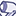# QlikView App Dev

Discussion Board for collaboration related to QlikView App Development.

Announcements
Skip the ticket, Chat with Qlik Support instead for instant assistance.
cancel
Showing results for
Did you mean:Contributor III

## Use Slider range in an expression

I am trying to get count with a date range slider.The slider is at the month-year level.

Dataset has begin and end date.

The condition is if the begin date (month-year) and end date (month-year) is within the range of the slider selection and I want to include the record in the count. Other wise not include in count.

The sample is attached.

1 Solution

Accepted SolutionsMaster III

Hi Joe,

try this

=Num(Count({<begindate={'>=\$(=Min(Date([Month Year])))'},enddate={'<=\$(=Max(Date([Month Year])))'}>} country),'###,##0')

Regards,

Antonio

3 RepliesMaster III

Hi Joe,

try this

=Num(Count({<begindate={'>=\$(=Min(Date([Month Year])))'},enddate={'<=\$(=Max(Date([Month Year])))'}>} country),'###,##0')

Regards,

AntonioContributor III
Author

Thanks.

How can I add one more condition into the above Expressions.

Like a column called 'approved' That has values 'Y' or 'N'. And I want to include only the approved ='Y' in the above count.Master III

=Num(Count({<begindate={'>=\$(=Min(Date([Month Year])))'},enddate={'<=\$(=Max(Date([Month Year])))'},approved={'Y'}>} country),'###,##0')Community Browser